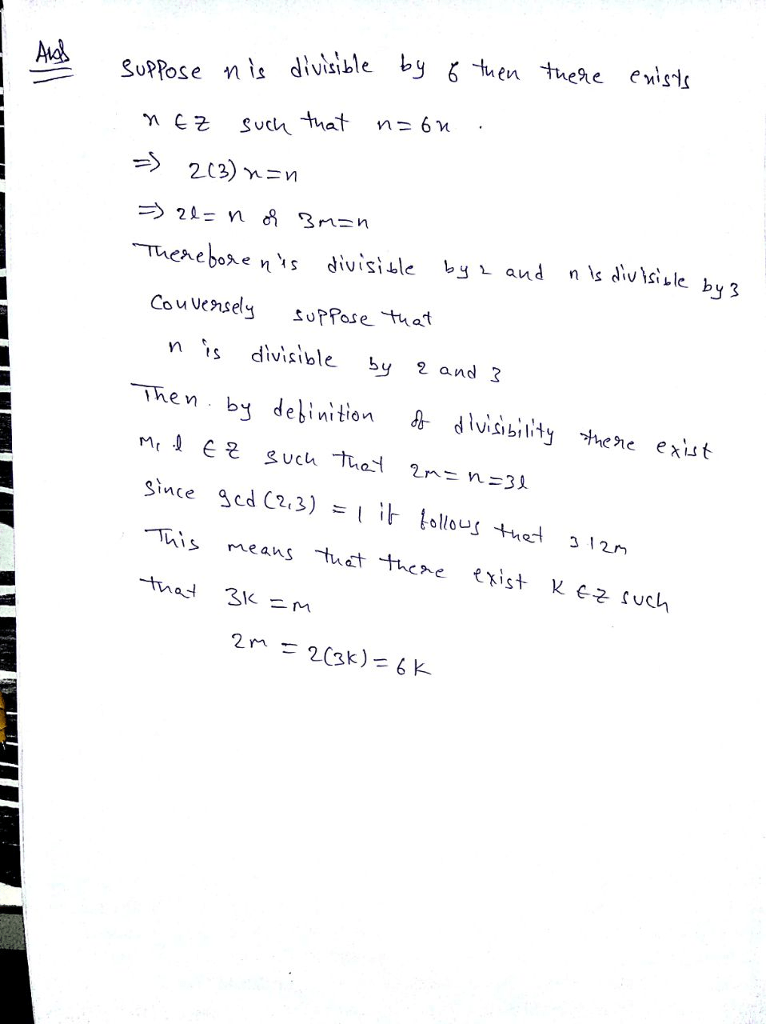# Give a formal proof that for all integers n, n is divisible by 6 if and only if n is divisible by 2 and n is divisible by 3.

Question-AnswerCategory: MathematicsGive a formal proof that for all integers n, n is divisible by 6 if and only if n is divisible by 2 and n is divisible by 3.

Give a formal proof that for all integers n, n is divisible by 6 if and only if n is divisible by 2 and n is divisible by 3.